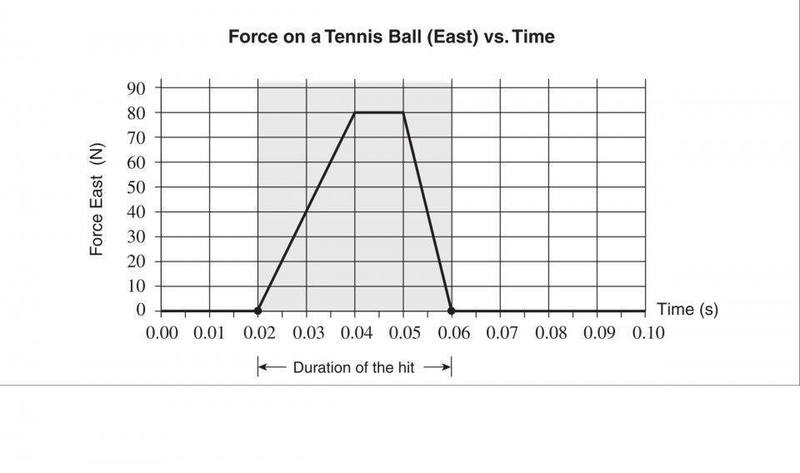# What is the magnitude of the final momentum?

## Homework Statement

A 0.057 kg tennis ball moving west at 24 m s is hit in the opposite direction east with a tennis racket. The force acting on the ball during the hit is shown in the graph below.What is the magnitude of the final momentum of the tennis ball?
A. 0.63 kgm s B. 1.37 kgm s C. 1.80 kgm s D. 3.37 kgm s

## Homework Equations

f(tf-ti)=m(vf-vi)= change in momentum=impulse[/B]

## The Attempt at a Solution

so I assumed that I could find final velocity going east by doing this

f ( tf - ti )= m ( vf - vi )

80 ( 0.06-0.02 ) = 0.057 ( vf - (-24) )
3.2 = 0.057 ( vf - (-24) )
56.14 = vf - (-24)
vf=32 m/s

so momentum right is (0.057)(32)=1.824 kg*ms

left is (0.057)(-24)= -1.368 kg*ms

final is 1.824 + (-1.368) = .456kg*ms to the right

this is incorrect so I'm not sure where i went wrong, i'm sure its simple but it escapes me.
thanks for any insight

haruspex
Homework Helper
Gold Member
2020 Award
The Force is not constant at 80 over the .04 seconds.

jbriggs444
Homework Helper
Impulse can be computed as the product of force multiplied by time for constant forces. If the force is varying, then things are not quite that simple. At a guess, you are not familiar with calculus and, in particular, with integrals. Let me give you a 30 second introduction to integrals...

Since force is not constant, do not treat the collision with the tennis racket as a single event. Instead, consider it as a sequence of smaller collisions, each for a much shorter time interval. If you make the time intervals short enough, the force during each interval will be almost constant. So you can multiply the duration of each of these mini-collisions by the force during that mini-collision and add them all up. That gives you an estimate of the total impulse. Graphically, what you are doing is dividing the area under the force-versus-time graph into a bunch of tall but slender rectangles and adding up the areas of all the rectangles.

If you let the width of the rectangles get smaller and smaller, the total area of the rectangles will come closer and closer to being the total area under the force versus time graph. This exact area is called the "integral of force over time" and is exactly equal to the impulse delivered by the collision.

You do not have to add up the areas of a bunch of rectangles to figure out the area under the curve. You should be able to figure out the area under the graph with some simple rules (e.g. the rules for computing the areas of rectangles and triangles)

thank you very much, so i got.

area under curve = 200
number of time intervals of that total = 4

200/4=50
50*0.04= 2
2/0.057=35.08
35.08-24=11.08
11.08*0.057=.632

is this what you meant?

jbriggs444
Homework Helper
thank you very much, so i got.

area under curve = 200
200 in units of graph squares. Always show your units when writing down numbers.

number of time intervals of that total = 4

200/4=50
50*0.04= 2
2/0.057=35.08
35.08-24=11.08
11.08*0.057=.632
Avoid putting numbers into equations. Put variables into equations. Substitute the numbers in at the end. That way those of us looking at your work have a better chance of understanding what you are trying to do.

You do not show your work computing the area under the curve but come up with a result of 200 graph square units. I'd expected some invocation of "1/2 base times height", "base times height" and "1/2 base times height" in your work product.

You divide by the duration of the collision -- 4 graph width units. That gives you the average force in graph height units -- 50 graph height units.

You convert this 50 graph height units to 50 Newtons and multiply by the elapsed time of .04 seconds. This gives you the average force multipled by the collision duration -- 2 Newton-seconds.

It would have been simpler if you had computed the area under the curve in Newton-seconds to start with. Then you would not have to uselessly divide by 4 and then multiply by 0.04 when all you are achieving is a unit conversion.

You divide this impulse by the mass of the tennis ball, 0.057 kg to get a change in velocity -- 35.08 meters/second

You assume a sign convention and subtract the initial velocity, 24 meters/second from the velocity change to get the final velocity -- 11.08 meters/second. You multiply by the mass of the tennis ball to get the resulting momentum -- 0.632 kg meters/second.

Rather than dividing by the mass of the tennis ball and then multiplying by the mass of the tennis ball, you could have worked with momenta directly.

is this what you meant?

Yes, more complicated than it had to be and less symbolic, but it seems correct.